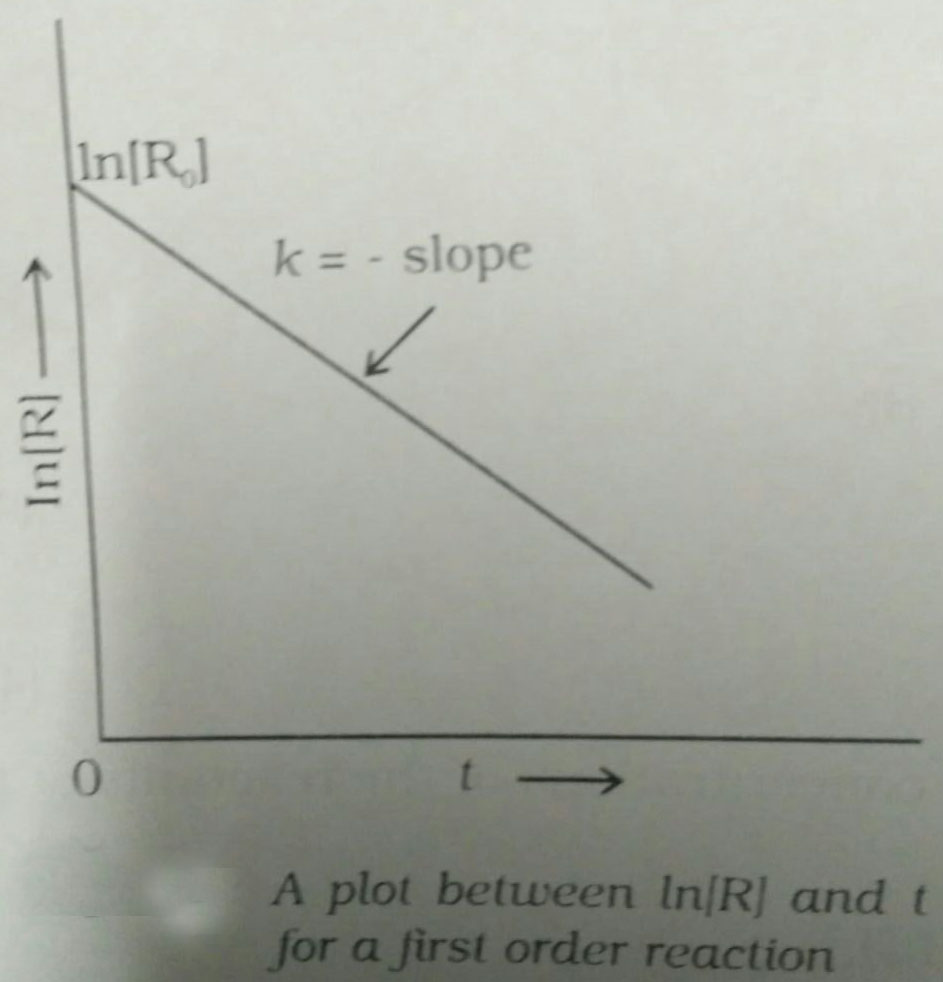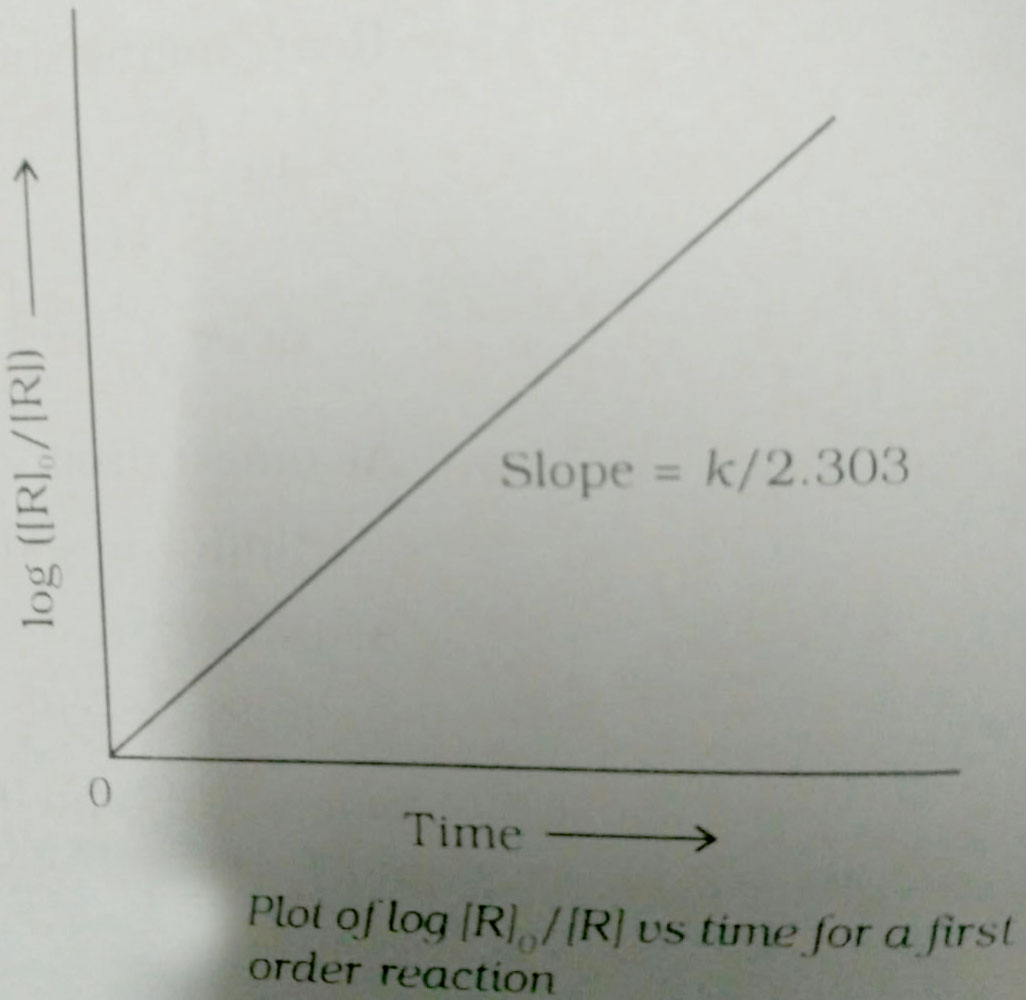Q

# Help me please, The half­-life of a radioactive isotope is three hours. If the initial mass of the iosotope were 256 g, the mass of it remaining undecayed after 18 hours would be

The half­-life of a radioactive isotope is three hours. If the initial mass of the iosotope were 256 g, the mass of it remaining undecayed after 18 hours would be

• Option 1)

4.0 g

• Option 2)

8.0 g

• Option 3)

12.0 g

• Option 4)

16.0 g.

344 Views

As we learnt in concept

First Order Reaction -

The rate of the reaction is proportional to the first power of the concentration of the reaction- wherein

Formula:

R            P

a                 0

a-x             x

[differentiate rate law]

Unit of

X = 4 gm

Option 1)

4.0 g

This option is correct.

Option 2)

8.0 g

This option is incorrect.

Option 3)

12.0 g

This option is incorrect.

Option 4)

16.0 g.

This option is incorrect.

Exams
Articles
Questions